Study Materials

# NCERT Solutions for Class 9th Mathematics

Page 2 of 4

## Chapter 7. Triangles

### Exercise 7.2

Chapter 7. Triangles

Exercise 7.2

Q1. In an isosceles triangle ABC, with AB = AC, the bisectors of ∠ B and ∠ C intersect each other at O. Join A to O. Show that :

(i) OB = OC (ii) AO bisects ∠ A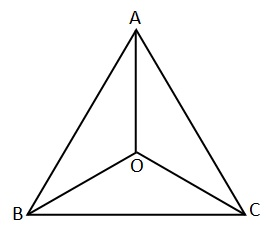Solution:

Given: In an isosceles triangle ABC, with AB = AC,  the bisectors of ∠ B and ∠ C intersect each other at O.

To prove:

(i) OB = OC

(ii) AO bisects ∠ A

Proof: In  ΔABC, We have:

AB = AC

∠ B = ∠ C [ opposite angle to the equal side ]

Or  1/2 ∠ B = 1/2 ∠C

So, ∠OBC = ∠OCB […1]

In D ABO and D ACO

AB = AC [given]

∠OBC = ∠OCB [from …1]

AO = AO [common]

By SAS Congruence Criterion Rule

DABO  DACO

OB = OC [ By CPCT ]

∠BAO = ∠CAO [ By CPCT ]

AO bisect ∠A.

Q2. In Δ ABC, AD is the perpendicular bisector of BC (see Fig. 7.30). Show that Δ ABC is an isosceles triangle in which AB = AC.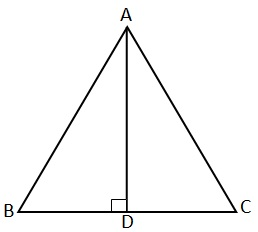Solution:

Given: In Δ ABC, AD is the perpendicular bisector of BC.

To prove: Δ ABC is an isosceles triangle in which AB = AC.

Proof: In Δ ABD and Δ ACD, we have

DB = DC [since D bisect BC]

By SAS Congruence Criterion Rule

Δ ABD  Δ ACD

AB =AC [CPCT]

Hence, Δ ABC is an isosceles triangle

Q3. ABC is an isosceles triangle in which altitudes BE and CF are drawn to equal sides AC and AB respectively (see Fig. 7.31). Show that these altitudes are equal.

Solution:

Given: ABC is an isosceles triangle in which BE ⊥ AC and CF ⊥ AB where AB = AC.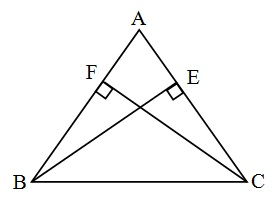To prove: BE = CF.

Proof:  Here, BE ⊥ AC and CF ⊥ AB  (Given)

In ΔABE and Δ ACF

∠ AEB = ∠ AFC  (90 Each)

∠ A = ∠ A       (Common)

AB = AC        (Given)

By ASA Congruency Criterion Rule

ΔABE    Δ ACF

BE = CF [ By CPCT ]

Q4. ABC is a triangle in which altitudes BE and CF to sides AC and AB are equal (see Fig. 7.32). Show that

(i) Δ ABE ≅ Δ ACF

(ii) AB = AC, i.e., ABC is an isosceles triangle.Solution:

Given: ABC is a triangle in which

BE ⊥ AC and CF ⊥ AB and BE = CF

To Prove :

(i) Δ ABE ≅ Δ ACF

(ii) AB = AC, i.e., ABC is an isosceles triangle.

Proof :

(i) In Δ ABE and Δ ACF

BE = CF           (Given)

∠ AEB = ∠ AFC     (90 Each)

∠ A = ∠ A         (Common)

Using ASA Congruency Property

Δ ABE ≅ Δ ACF   Proved I

(ii)            AB = AC  [By CPCT

Therefore, ABC is an isosceles triangle.

Q5. ABC and DBC are two isosceles triangles on the same base BC (see Fig. 7.33). Show that ∠ ABD = ∠ ACD.

Solution: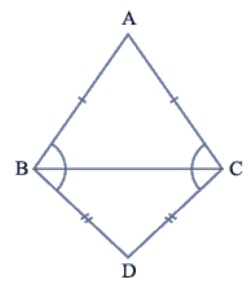Given : ABC and DBC are two isosceles triangles on the same base BC.

To prove : ∠ ABD = ∠ ACD

Proof : ABC is an isosceles triangle in which

AB = AC      (Given)

∴      ∠ ABC = ∠ ACB           .......... (1)

(Opposite angles of equal sides)

Similarily,

BCD is also an isosceles triangle.

BD = CD      (Given)

∴      ∠ DBC = ∠ DCB           .......... (2)

(Opposite angles of equal sides)

∠ ABC + ∠ DBC = ∠ ACB + ∠ DCB

Or,           ∠ ABD = ∠ ACD       Proved

Q6. ΔABC is an isosceles triangle in which AB = AC. Side BA is produced to D such that AD = AB (see Fig. 7.34). Show that ∠ BCD is a right angle.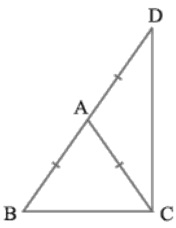Solution :

Given: ΔABC is an isosceles triangle in which AB = AC.

Side BA is produced to D such that AD = AB

To prove : ∠ BCD = 90

Proof :

AB = AC      .............. (1)  (Given)

And,   AB = AD      .............. (2)  (Given)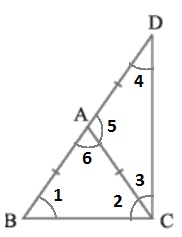From equation (1) and (2) we have

∴      ∠3 = ∠4     .... (4) (Opposite angles of equal sides)

Now, AB = AC    from (1)

∴       ∠1 = ∠2     .... (5) (Opposite angles of equal sides)

In ΔABC,

Exterior ∠5 = ∠1 + ∠2

Or,         ∠5 = ∠2 + ∠2       from (5)

Or,         ∠5 = 2∠2    ....... (6)

Similarily,

Exterior ∠6 = ∠3 + ∠4

Or,         ∠6 = 2∠3      from (7)

∠5 + ∠6  = 2∠2 + 2∠3

∠5 + ∠6  = 2(∠2 + ∠3)

Or,     180० = 2(∠2 + ∠3)   [ ∵ ∠BAC + ∠DAC  = 180० ]

Or,    ∠2 + ∠3 = 180० / 2

Or,     ∠BCD = 90०      Proved

Q7. ABC is a right angled triangle in which ∠ A = 90° and AB = AC. Find ∠ B and ∠ C.

Solution: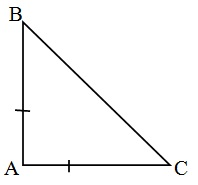Given : ABC is a right angled triangle in which

∠ A = 90° and AB = AC.

To Find : ∠B and C

AB = AC          (Given)

∴            ∠B = ∠C      ............(1)

(Opposite angles of equal sides)

In triangle ABC,

∠A + ∠B + ∠C = 180०   (Angle sum property)

90° + ∠B + ∠B = 180०   Using equation (1)

∠B = 180- 90°

2 ∠B = 90°

∠B =  90°/ 2

∠B =  45°

∴ ∠B = 45° and ∠C =  45°

Q8. Show that the angles of an equilateral triangle are 60° each. ⊥

Solution: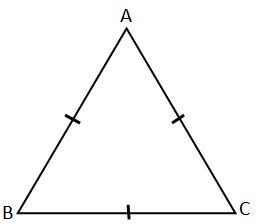Given: ABC is a equilateral triangle, in which

AB = BC = AC

To Prove :

∠A = ∠B = ∠C = 60°

Proof :

AB = AC      (Given)

∠B = ∠C     ....................... (1)   [opposite angle of equal sides]

AB = BC       (Given)

∠A = ∠C     ....................... (2)   [opposite angle of equal sides]

AC = BC       (Given)

∠A = ∠B     ....................... (3)   [opposite angle of equal sides]

From equation (1), (2) and (3) we have

∠A = ∠B = ∠C     .............. (4)

In triangle ABC

∠A + ∠B + ∠C = 180°

∠A + ∠A + ∠A = 180°

3 ∠A = 180°

∠A = 180°/3

∠A = 60°

∴ ∠A = ∠B = ∠C = 60°

Page 2 of 4

Chapter Contents: